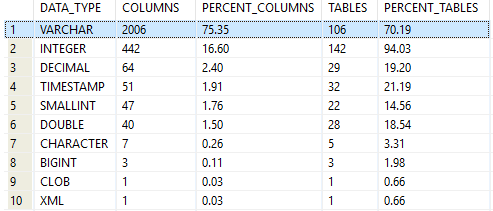## Find most used data type in IBM Db2 database

Article for:

Query below returns data types used in a database ordered by the number of their occurance.

## Query

``````select col.typename as data_type,
count(*) as columns,
cast(100.0 *
count(*) /
(select count(*) from syscat.tables tab
inner join syscat.columns col
on col.tabschema = tab.tabschema
and col.tabname = tab.tabname
where tab.type = 'T'
and tab.tabschema not like 'SYS%')
as decimal (14,2))  as percent_columns,
count(distinct tab.tabschema concat '.' concat tab.tabname) as tables,
cast(100.0 *
count(distinct tab.tabschema concat '.' concat tab.tabname) /
(select count(*) from syscat.tables
where type = 'T' and tabschema not like 'SYS%')
as decimal (14,2)) as percent_tables
from syscat.tables tab
inner join syscat.columns col
on col.tabschema = tab.tabschema and col.tabname = tab.tabname
where tab.type = 'T'
and tab.tabschema not like 'SYS%'
group by col.typename
order by count(*) desc
``````

## Columns

• data_type - built in or user data type without length or precision, e.g. int, varchar or date
• columns - number of columns in a database with this data type
• percent_columns - percentage of columns with this data type. Rows add up to 100%
• tables - number of tables in a database with this data type
• percent_tables - percentage of tables with columns with this data type.

## Rows

• One row represents one data type
• Scope of rows: all data types used in a database
• Ordered by number of columns descending

## Sample results

Those results show data types used in GSDB database. As you can see most popular data type is VARCHAR - it is used in 2006 columns which is 75.36% of all columns in 106 tables (which 70.19% of all tables).